# COPRIME3 - Editorial

Nice problem, learnt something new.

1 Like

I am trying to explain it in lengthy way but this is very very very helpful and easy to understand.

Before explaining solution I want to make you understand something that will be very helpful for this problem. You will think why I am explaining very easy concepts, there is only one reason — I want to make my editorial understand for everyone. Everyone means everyone — who are new to the programming.

1. What is GCD?

Full form for GCD is Greatest Common Divisor. Let’s understand this concept by taking an example.

Let say a = 10, b = 20. What is the GCD of a, b?

Divisors for “a” are [1,2,5,10] and Divisors for “b” are [1,2,4,5,10,20]. What is the greatest common divisor between these two — “10”. So the answer is 10. This is very basic concept.

1. What are co-prime numbers?

Definition: Two numbers are co-prime if GCD of those two numbers are 1 .

Let’s understand this concept by taking an example.

Let say a = 5, b = 7. These two numbers are co-prime or not? Divisors for “a” are [1,5] and Divisors for “b” are [1,7]. So GCD(a, b) = 1 . So, these two numbers are co-prime.

Let say a = 5, b = 10. These two numbers are co-prime or not? Divisors for “a” are [1,5] and Divisors for “b” are [1,5,10]. So GCD(a, b) = 5 . So, these two numbers are not co-prime.

Problem Statement: Given a sequence [A1, A2, …, AN]. Count number of triples (i, j, k) such that 1 ≤ i < j < k ≤ N and GCD(Ai, Aj, Ak) = 1.

So in brief, we have to find number of co-prime triples in an array.

Now, what is the first thing in your mind when you want to solve this problem? I think check for every number in array, check whether triple have GCD 1, if GCD is 1 for triple then increment count. Repeat this process until last element of array and print count.

Yes this is nice approach, But I have better approach for this problem than this. Now let’s understand better approach by taking an example. First we will find number of pairs with 2 elements which are co-prime. Don’t forget this.arr = [1,2,3,4,5,6,7,8,9,10,11,12,13,14,15,16,17,18,19,20]

Now we will write multiples of each number till last element and also count how many multiples are there for each multiple in array.

multiples of 1 = [1,2,3,4,5,6,7,8,9,10,11,12,13,14,15,16,17,18,19,20] (20)

multiples of 2 = [2,4,6,8,10,12,14,16,18,20] (10)

multiples of 3 = [3,6,9,12,15,18] (6)

multiples of 4 = [4,8,12,16,20] (5)

multiples of 5 = [5,10,15,20] (4)

multiples of 6 = [6,12,18] (3)

multiples of 7 = [7,14] (2)

multiples of 8 = [8,16] (2)

multiples of 9 = [9,18] (2)

multiples of 10 = [10,20] (2)

multiple of 11 =  (1)

multiple of 12 =  (1)

multiple of 13 =  (1)

multiple of 14 =  (1)

multiple of 15 =  (1)

multiple of 16 =  (1)

multiple of 17 =  (1)

multiple of 18 =  (1)

multiple of 19 =  (1)

multiple of 20=  (1)

Do you understand the concept? If not then it’s fine, if yes then it’s very fine. I am here for you.

Here what is the meaning of Multiples of 1?

• This will give you list of numbers whose GCD = multiple of 1 for any pair of two numbers in list.

Let’s understand above statement by one of the multiple.

multiples of 2 = [2,4,6,8,10,12,14,16,18,20] (10)

Here count is 10 for array for number of multiples of 2. This is the list of numbers whose GCD = multiple of 2 for any pair of two numbers in the list.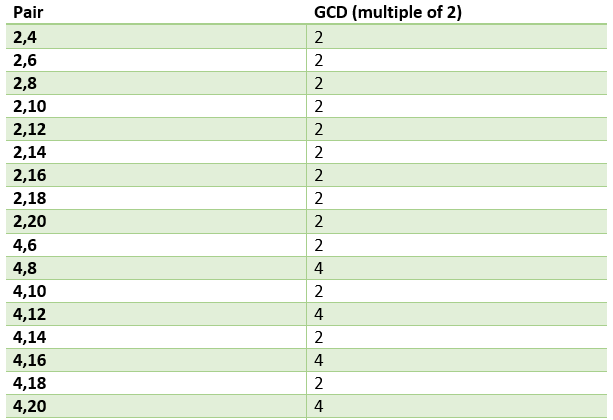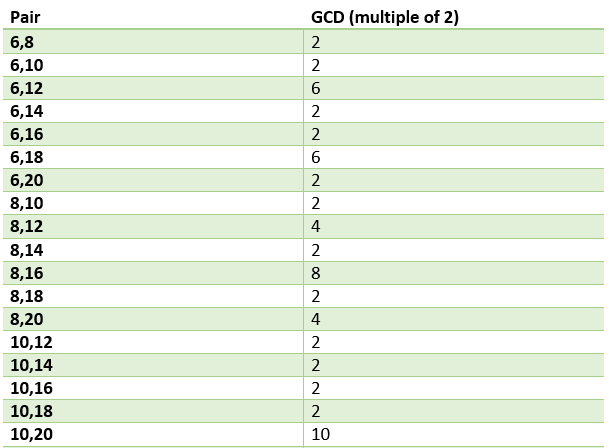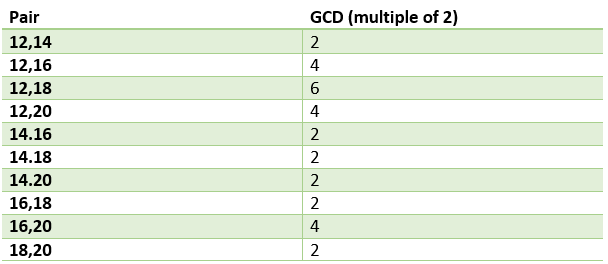Here you can see that GCD for all pairs is multiple of 2, because they are multiple for 2. This statement is true for all multiples(1 to 20) that we have defined above.

Now my question is how to find count of number of pairs which have GCD 2. Here from above table we can count that number of pairs are 45 . Yes, that is the count. Now next thing is how to find this count.

As we have 10 multiples of number 2. Here all numbers are multiple of 2. In how many ways we can select/choose 2 elements from these 10 elements? I think you have got me. In other words how many combinations of 2 elements to choose if we have 10 elements. This is combination thing. You can find this by (nCr) formula.

Here n = 10, r = 2. So, 10C2 = (10!)/((10–2)!*(2!)) = 45. Here is the link if you want to understand combination thing in deep. https://www.mathwords.com/c/combination_formula.htm

Let’s make out formula for Nc2. (N!)/((N-2)!2!) -> (N(N-1)*(N-2)!)/((N-2)!2!) -> (N(N-1))/2.

Now we will calculate this for every count, so here we are counting number of pairs with GCD of multiple of “x”.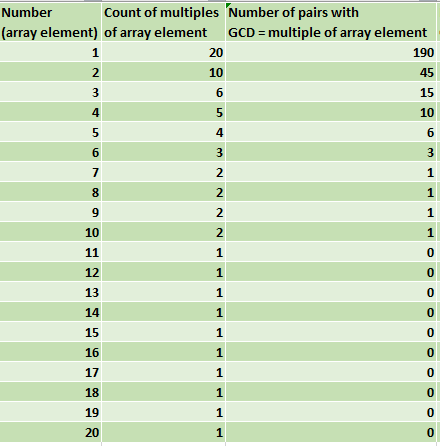Have you got now what I am trying to say? If yes then It is very good, if no then also it’s fine because I am here for you.

Now it is the table for number of pairs with GCD = multiple of each element of array . Now Let me tell you one fact from this table. Let say we want to find number of pairs with GCD = EXACTLY 2 . How can we find this from table? Here Number 2 contains number of pairs with GCD of multiple of 2. Let me tell you in another words, number 2(2nd row) contains number of pairs with GCD = 2,4,6,8,10,12,14,16,18,20). How can we find GCD = exactly 2. Simple,

We need to remove number of pairs with GCD = exactly 4,6,8,10,12,14,16,18,20. Here we will go backwards. Because we have to find number of pairs with exactly array element. So number of pairs with GCD exactly 20 = (number of pairs with GCD exactly 20-number of pairs with GCD exactly 40 etc.) = 0

GCD exactly 19 = (number of pairs with GCD exactly 19-number of pairs with GCD exactly 38 etc.) = 0

And so on. Now If we don’t go backwards then we will subtract GCD multiple of array element. Here we are finding pairs with exact GCD.

Multiple of 2 except 2. So, GCD = exactly 2 -> 45(GCD of multiple of 2)-9(GCD exactly 4)-3(GCD exactly 6)-1(GCD exactly 8)-1(GCD exactly 10)-0(GCD exactly 12)-0(GCD exactly 14)-0(GCD exactly 16)-0(GCD exactly 18)-0(GCD exactly 20)=31. There are 31 pairs which have GCD exactly 2.

Why are we removing this?

Here 2 will contain number of pairs with GCD 2,4,6,8,10,12,14,16,18,20.

3 will contain number of pairs with GCD 3,6,9,12,15,18.

4 will contain number of pairs with GCD 4,8,12,16,20.

So we are removing other pairs for each array element except current element.

So, from this table we can say that in our example number of pairs(2 elements) with GCD = 1(co-prime) are 127 .

Now focus to our main problem, where we have to find number of triplets with GCD = 1(co-prime). Only one thing will change here. Instead of nC2, we will take nC3. Because we have to select/choose 3 elements from these 10 elements?

Here is the formula.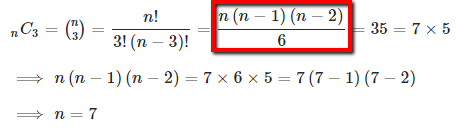Formula for nC3

Here is the table for co-prime triples.

So, answer here for number of pairs with GCD exactly 1 is 997 .

Here is the solution link for the problem.

If you find this editorial helpful, please give heart for it as I have put so much hard work for it.

Thank you.

21 Likes

Thanks for the explanation. : - )

1 Like